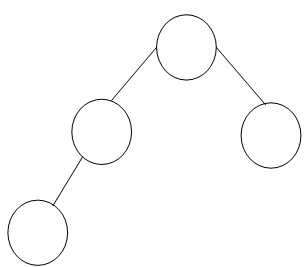## Avl trees, Data Structure & Algorithms

Assignment Help:

An AVL tree is a binary search tree that has the given properties:

• The sub-tree of each of the node differs in height through at most one.
• Each sub tree will be an AVL tree.

Figure illustrated an AVL tree.Figure: Balance requirement for AVL tree: the left & right sub tree differ by at most one in height

AVL stands for the names of G.M. Adelson - Velskii & E.M. Landis, two Russian mathematicians, who came up along with this method of keeping the tree balanced.

An AVL tree is a binary search tree that has the balance property and additionally to its key, each of the node stores an additional piece of information: the current balance of its subtree. The three possibilities are following:

• Left - HIGH (balance factor -1)

The left child contain a height which is greater than the right child by 1.

• BALANCED (balance factor 0)

Both of children have the same height

• RIGHT - HIGH (balance factor +1)

The right child contains a height that is greater by 1.

An AVL tree that remains balanced guarantees O(log n) search time, even into the worst case. Here, n refer to the number of nodes. The AVL data structure gain this property by placing limitation on the difference in heights among the sub- trees of given node and rebalancing the tree even if it violates these limitation.

#### Related Discussions:- Avl trees

representation of links list in memory

#### Undirected graph, Graphs are data structures which consist of a set of vert...

Graphs are data structures which consist of a set of vertices & a set of edges which connect the vertices. A graph where the edges are directed is called directed graph. Or else, i

#### Algorithm for similar binary tree, Q. The two Binary Trees are said to be s...

Q. The two Binary Trees are said to be similar if they are both empty or if they are both non- empty and left and right sub trees are similar. Write down an algorithm to determine

#### What are the languages which support assertions, What are the languages whi...

What are the languages which support assertions Languages which support assertions often provide different levels of support. For instance, Java has an assert statement which t

#### Algorithm that inputs the codes for all items in stock, A shop sells books,...

A shop sells books, magazines and maps. Every item is identified by a unique 4 - digit code. All books have a code which starts with 1, all maps have a code starting with 2 and all

#### Define a b-tree, Define a B-Tree Justas AVL trees are balanced binary s...

Define a B-Tree Justas AVL trees are balanced binary search trees, B-trees are balanced M-way search trees. A B-Tree of order M is either the empty tree or it is an M-way searc

#### Implementation of stack, Implementation of Stack :- Stacks can be execu...

Implementation of Stack :- Stacks can be executed in the 2 ways: a)  Arrays b)  Linked List

#### What is quick sort, What is quick sort?   Answer Quick sort is on...

What is quick sort?   Answer Quick sort is one of the fastest sorting algorithm used for sorting a list. A pivot point is chosen. Remaining elements are divided or portio

#### Binary search tree, write an algorithm to delete an element x from binary...

write an algorithm to delete an element x from binary search with time complex

#### C++ function, Write c++ function to traverse the threaded binary tree in in...

Write c++ function to traverse the threaded binary tree in inorder traversal